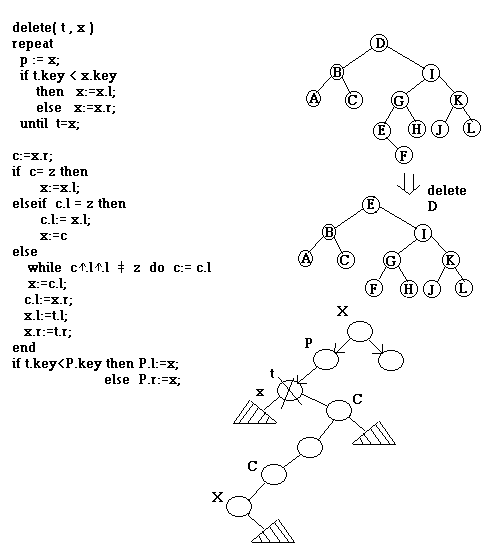Searching

•  retrieve records with Keys matching a given Key
• dictionary , symbol tables

• Operations: search
Insert
delete
join
sort
Sequential search:
• array : E X A M P L E       T = N : unsuccessful

•                                             =  N/2 : successful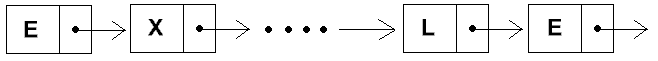• most frequently accessed records go first
• self - organized:
E X A M P L E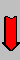Search M
M E X A P L ESearch L
L M E X A P EAssume locality of reference for search keys
Binary search ( sorted file ):

1     2      3    4    5     6    7     8     9    10   11   12   13   14  15   16   17
--------------------------------------------------------------------------------------------
A    A    A    C    E     E    E    G    H     I     L    M    N    P    R    S    X
A    A    A    C    E     E    E    G    H     I     L    M    N    P    R    S    X
I     L    M    N    P    R    S    X
P    R   S    X
S    X

T = lg N

Binary search Tree ( dynamic ) :

T = lg N ( ave. )
N     ( worst )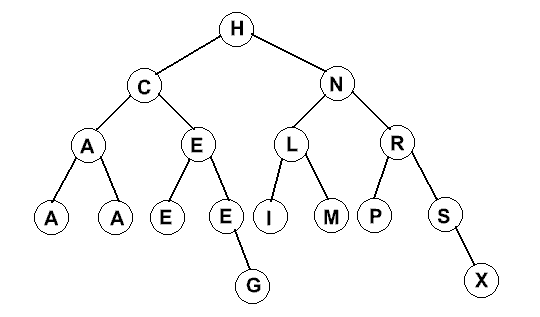Sort
x -> root
treeprint(x)                             /*  in-order traversal  */
if  x !=  nil  then {
treeprint (xup. left);
printNode(x);
treeprint(xup. right);
}

Interpolation search: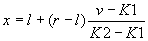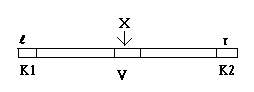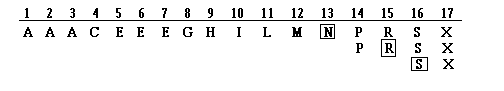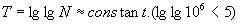Deletion :